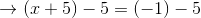# High School Math : Understanding Negative Exponents

## Example Questions

### Example Question #1 : Exponents

Which of the following is equivalent to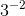?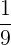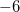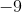Explanation:

By definition,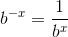.

In our problem,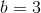andThen, we have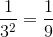.

### Example Question #2 : Exponents

Solve for: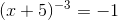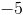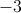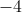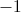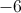Explanation:

Raise both sides of the equation to the inverse power ofto cancel the exponent on the left hand side of the equation.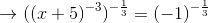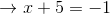Subtractfrom both sides: